Dominoes in Squares
The following pictures show n dominoes with short sides 1, 2, . . . n packed inside the smallest square (of side length s). All of these are probably optimal, except for possibly n=20.

 1.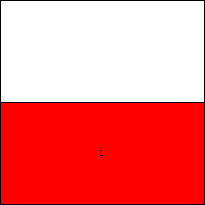s = 2Trivial. 2.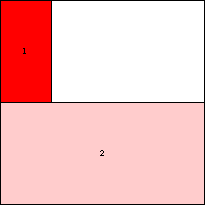s = 4Trivial. 3.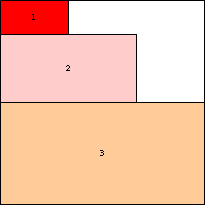s = 6Trivial. 4.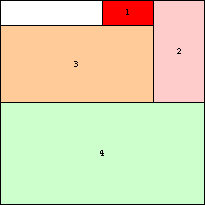s = 8Trivial. 5.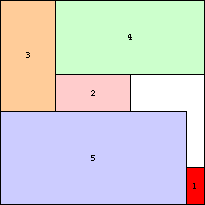s = 11Found by Erich Friedman in 1998. 6.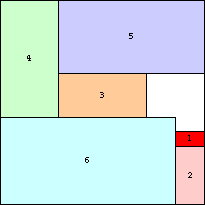s = 14Found by Erich Friedman in 1998. 7.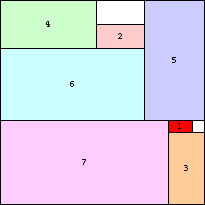s = 17Found by Erich Friedman in 1998. 8.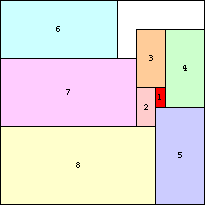s = 21Found by Erich Friedman in 1998. 9.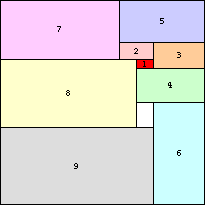s = 24Found by Erich Friedman in 1998. 10.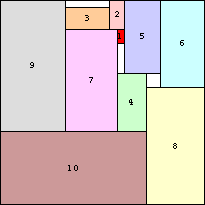s = 28Found by Erich Friedman in 1998. 11.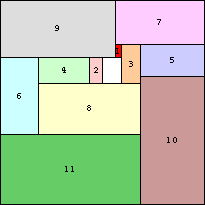s = 32Found by Erich Friedman in 1998. 12.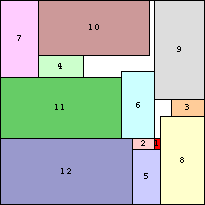s = 37Found by Erich Friedman in 1998. 13.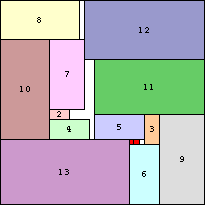s = 41Found by Erich Friedman in 1998. 14.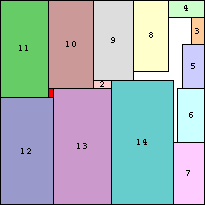s = 46Found by Erich Friedman in 2004. 15.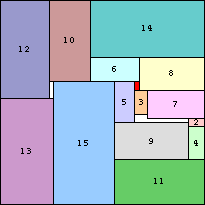s = 50Found by Erich Friedman in 2004. 16.s = 55Found by Erich Friedman in 2004. 17.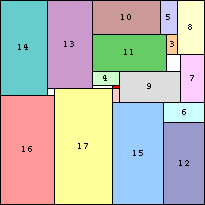s = 60Found by Erich Friedman in 2004. 18.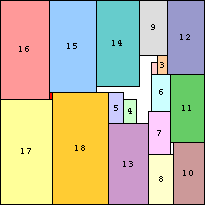s = 66Found by Erich Friedman in 2004. 19.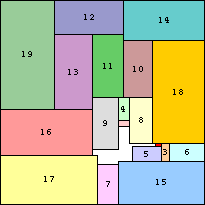s = 71Found by Erich Friedman in 2004. 20.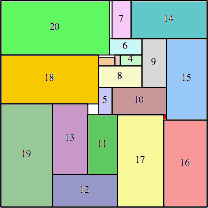s = 76Found by Maurizio Morandi in 2014. 21.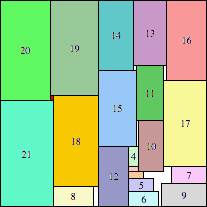s = 82Found by Erich Friedman in 2014. 22.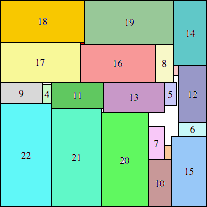s = 88Found by Erich Friedman in 2014. 23.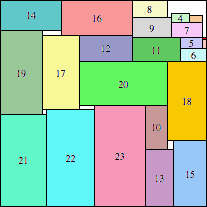s = 94Found by Erich Friedman in 2014. 24.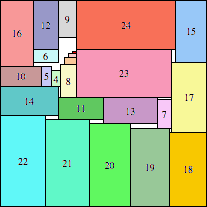s = 100Found by Erich Friedman in 2014.